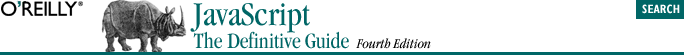home | O'Reilly's CD bookshelfs | FreeBSD | Linux | Cisco | Cisco Exam## 5.8. Bitwise Operators

Bitwise AND (&)
The & operator performs a Boolean AND operation on each bit of its integer arguments. A bit is set in the result only if the corresponding bit is set in both operands. For example, 0x1234 & 0x00FF evaluates to 0x0034.

Bitwise OR (|)
The | operator performs a Boolean OR operation on each bit of its integer arguments. A bit is set in the result if the corresponding bit is set in one or both of the operands. For example, 9 | 10 evaluates to 11.

Bitwise XOR (^)
The ^ operator performs a Boolean exclusive OR operation on each bit of its integer arguments. Exclusive OR means that either operand one is true or operand two is true, but not both. A bit is set in this operation's result if a corresponding bit is set in one (but not both) of the two operands. For example, 9 ^ 10 evaluates to 3.

Bitwise NOT (~)
The ~ operator is a unary operator that appears before its single integer argument. It operates by reversing all bits in the operand. Because of the way signed integers are represented in JavaScript, applying the ~ operator to a value is equivalent to changing its sign and subtracting 1. For example ~0x0f evaluates to 0xfffffff0, or -16.

Shift left (<<)
The << operator moves all bits in its first operand to the left by the number of places specified in the second operand, which should be an integer between 0 and 31. For example, in the operation a << 1, the first bit (the ones bit) of a becomes the second bit (the twos bit), the second bit of a becomes the third, etc. A zero is used for the new first bit, and the value of the 32nd bit is lost. Shifting a value left by one position is equivalent to multiplying by 2, shifting two positions is equivalent to multiplying by 4, etc. For example, 7 << 1 evaluates to 14.

Shift right with sign (>>)
The >> operator moves all bits in its first operand to the right by the number of places specified in the second operand (an integer between and 31). Bits that are shifted off the right are lost. The bits filled in on the left depend on the sign bit of the original operand, in order to preserve the sign of the result. If the first operand is positive, the result has zeros placed in the high bits; if the first operand is negative, the result has ones placed in the high bits. Shifting a value right one place is equivalent to dividing by 2 (discarding the remainder), shifting right two places is equivalent to integer division by 4, and so on. For example, 7 >> 1 evaluates to 3 and -7 >> 1 evaluates to -4.

Shift right with zero fill (>>>)
The >>> operator is just like the >> operator, except that the bits shifted in on the left are always zero, regardless of the sign of the first operand. For example, -1 >> 4 evaluates to -1, but -1 >>> 4 evaluates to 268435455 (0x0fffffff).